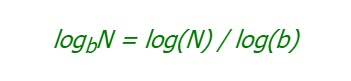# Perl | log() Function

log() function in Perl returns the natural logarithm of value passed to it. Returns \$_ if called without passing a value. log() function can be used to find the log of any base by using the formula:Syntax: log(value)

Parameter:
value: Number of which log is to be calculated

Returns:
Floating point number in scalar context

Example 1:

 `#!/usr/bin/perl -w ` ` `  `# Calculating log of base 10  ` `# using log function ` `print` `"log10(2): "``, log10(2), ``"\n"``; ` `print` `"log10(7): "``, log10(7), ``"\n"``; ` `print` `"log10(9): "``, log10(9), ``"\n"``; ` ` `  `# Function for log10 calculator ` `sub` `log10  ` `{ ` `    ``my` `\$n` `= ``shift``; ` `     `  `    ``# using pre-defined log function ` `    ``return` `log``(``\$n``) / ``log``(10); ` `} `

Output:

```log10(2): 0.301029995663981
log10(7): 0.845098040014257
log10(9): 0.954242509439325
```

Example 2:

 `#!/usr/bin/perl -w ` ` `  `# Calculating log of different  ` `# base using log function ` `print` `"log3(2): "``, log3(2), ``"\n"``; ` `print` `"log5(7): "``, log5(7), ``"\n"``; ` `print` `"log2(9): "``, log2(9), ``"\n"``; ` ` `  `# Function for log3 calculator ` `sub` `log3  ` `{ ` `    ``my` `\$n` `= ``shift``; ` `     `  `    ``# using pre-defined log function ` `    ``return` `log``(``\$n``) / ``log``(3); ` `} ` ` `  `# Function for log5 calculator ` `sub` `log5 ` `{ ` `    ``my` `\$n` `= ``shift``; ` `     `  `    ``# using pre-defined log function ` `    ``return` `log``(``\$n``) / ``log``(5); ` `} ` ` `  `# Function for log2 calculator ` `sub` `log2  ` `{ ` `    ``my` `\$n` `= ``shift``; ` `     `  `    ``# using pre-defined log function ` `    ``return` `log``(``\$n``) / ``log``(2); ` `} `

Output:

```log3(2): 0.630929753571457
log5(7): 1.20906195512217
log2(9): 3.16992500144231
```

My Personal Notes arrow_drop_upCheck out this Author's contributed articles.

If you like GeeksforGeeks and would like to contribute, you can also write an article using contribute.geeksforgeeks.org or mail your article to contribute@geeksforgeeks.org. See your article appearing on the GeeksforGeeks main page and help other Geeks.

Please Improve this article if you find anything incorrect by clicking on the "Improve Article" button below.

Article Tags :
Practice Tags :

Be the First to upvote.

Please write to us at contribute@geeksforgeeks.org to report any issue with the above content.